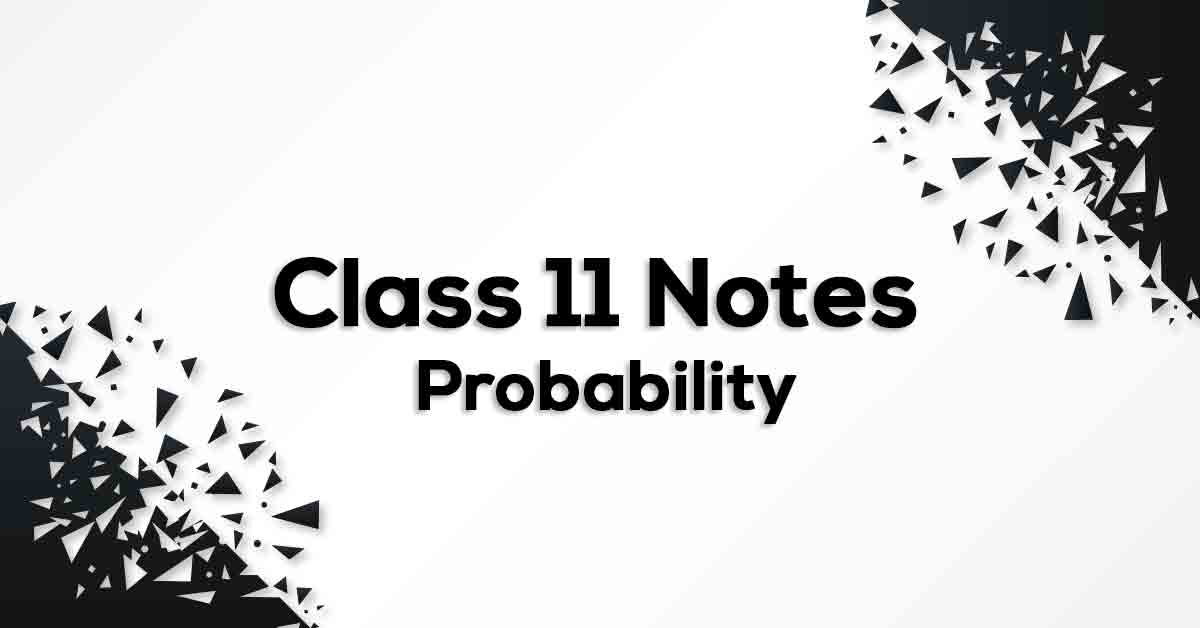# Probability Class 11 Formulas & Notes## Class 11 Math Chapter 16 Probability Formulae - PDF Download

Probability Class 11 Formulas & Notes are cumulated by our panel of highly experienced teachers to provide the students with effective exam preparation. The pdf not only includes the list of formulae but also offer students with the summary of the chapter, important points to remember and detailed explanation of important concepts and derivations for better understanding and retaining of the chapter. Going through these Probability Class 11 Formulas & Notes would easily help students to grasp challenging concepts which would ultimately lead them to score maximum marks in their exams.

### Understanding Probability.

Probability is the measure of the likeliness that an event will occur. Probability is quantified as a number between 0 and 1 (where 0 indicates impossibility and 1 indicates certainty). In mathematics, when next outcome of the experiment cannot be determined then we say it is a random experiment. A trial is an action which results in one or several outcomes, for example each toss of the coin and each throw of the die are called trials.

Learn more about trails, experiments and events in Probability Class 11 Formulas & Notes pdf.